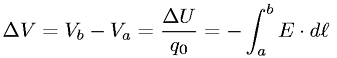Equations > Physics > Electricity and Magnetism > Change in potential

### Change in potentialLatex Code:

MathML Code:

 $\Delta V={V}_{b}-{V}_{a}=\frac\Delta U{q}_{0}=-{\int }_{a}^{b}E·d\ell$

MathType 5.0: